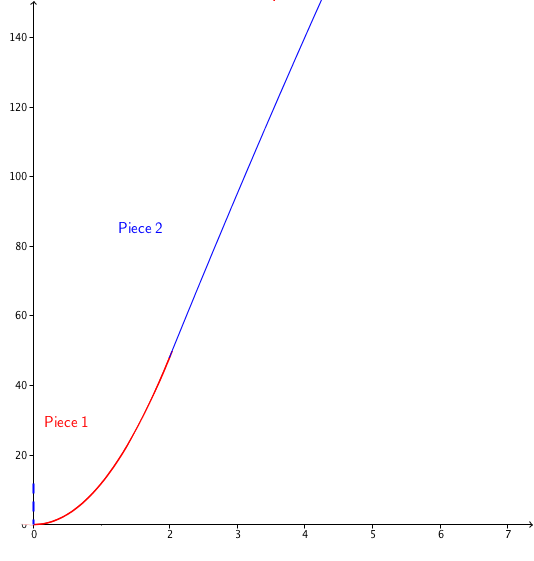### Home > APCALC > Chapter 1 > Lesson 1.4.4 > Problem1-184

1-184.

As a cheetah runs, its velocity is given by $v ( t ) = \left\{ \begin{array} { c c } { 12 t ^ { 2 } } & { \text { for } 0 \leq t \leq 2 } \\ { - t ^ { 2 } + 52 t - 52 } & { \text { for } t > 2 } \end{array} \right.$  where $v\left(t\right)$ is measured in feet per second.

1. Sketch a graph of the cheetah’s velocity.2. Approximately how fast is the cheetah running at $t = 1$ second? How did you get your answer?

This is a velocity function. So, what is the $y$ value at $x = 1$?

3. To catch prey, such as an antelope, the cheetah runs for $3$ seconds. Approximately how far does the cheetah need to run to catch its prey? Describe your method.

Use geometry to approximate the area under the curve.

Trapezoid Method $107.5$ ft
Midpoint Method $101.75$ ft
There are other valid geometric methods.

4. Annalou the Antelope standing $50$ feet north of the Great Pond. The cheetah spots Annalou and runs south in a direct line towards her, catching her in exactly $3$ seconds. What was the cheetah’s initial position relative to the pond?

Your answer in part (c) is an approximation of how far the cheetah traveled in $3$ seconds. At $t = 0$, Annalou was only $50$ feet north of the pond. Where was the cheetah?